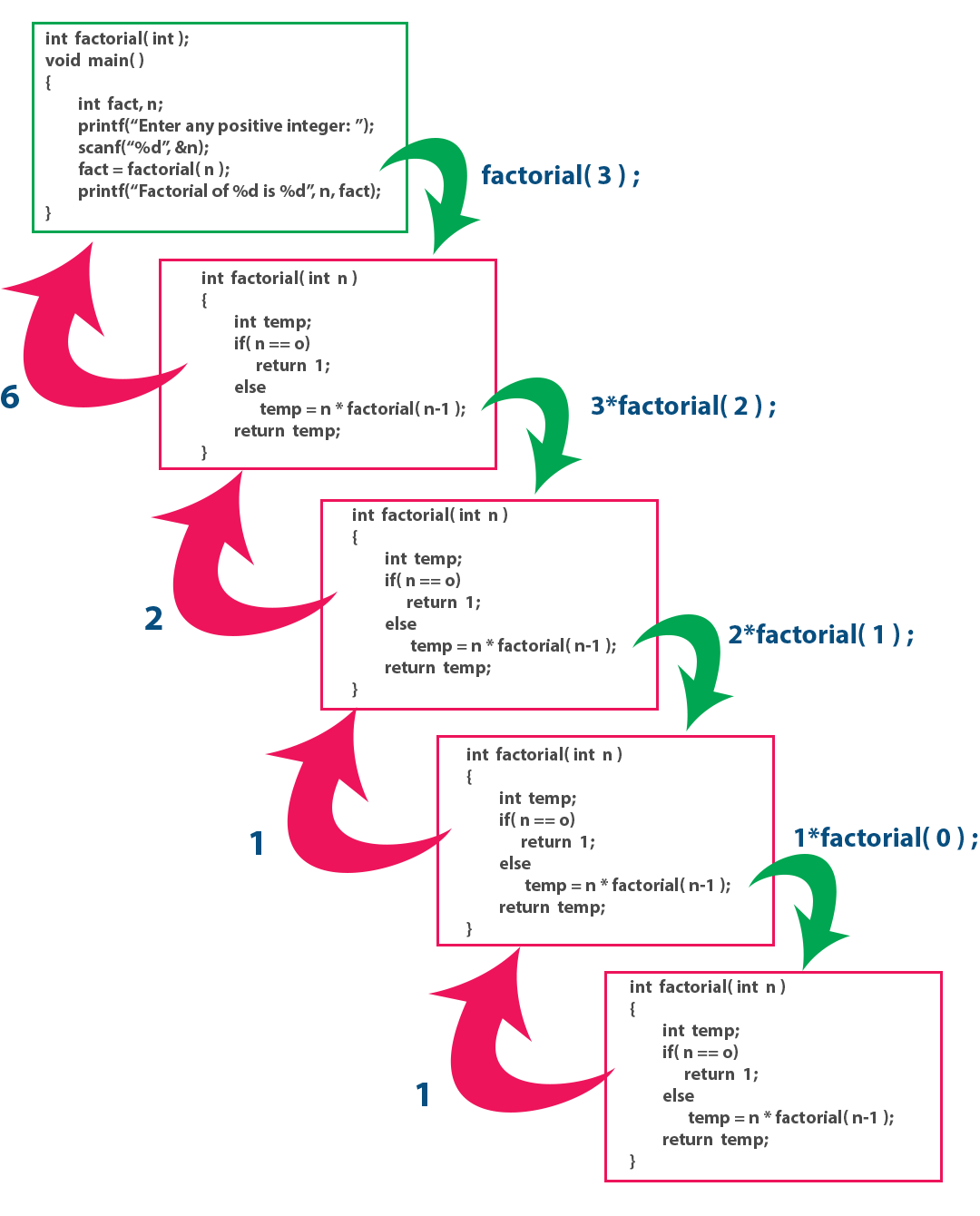The perfect place for easy learning...

×

# Recursive Functions in C

In C programming language, function calls can be made from the main() function, other functions or from the same function itself. The recursive function is defined as follows...

A function called by itself is called recursive function.

The recursive functions should be used very carefully because, when a function called by itself it enters into the infinite loop. And when a function enters into the infinite loop, the function execution never gets completed. We should define the condition to exit from the function call so that the recursive function gets terminated.

When a function is called by itself, the first call remains under execution till the last call gets invoked. Every time when a function call is invoked, the function returns the execution control to the previous function call.

# Example Program

``````#include<stdio.h>
#include<conio.h>

int  factorial( int ) ;
int main()
{
int  fact, n ;
printf("Enter any positive integer: ") ;
scanf("%d", &n) ;
fact = factorial( n ) ;
printf("\nFactorial of %d is %d\n", n, fact) ;
return 0;
}
int  factorial( int  n )
{
int  temp ;
if( n == 0)
return  1 ;
else
temp = n * factorial( n-1 ) ; // recursive function call
return  temp ;
}```
```

Output:In the above example program, the factorial() function call is initiated from main() function with the value 3. Inside the factorial() function, the function calls factorial(2), factorial(1) and factorial(0) are called recursively. In this program execution process, the function call factorial(3) remains under execution till the execution of function calls factorial(2), factorial(1) and factorial(0) gets completed. Similarly the function call factorial(2) remains under execution till the execution of function calls factorial(1) and factorial(0) gets completed. In the same way the function call factorial(1) remains under execution till the execution of function call factorial(0) gets completed. The complete execution process of the above program is shown in the following figure...